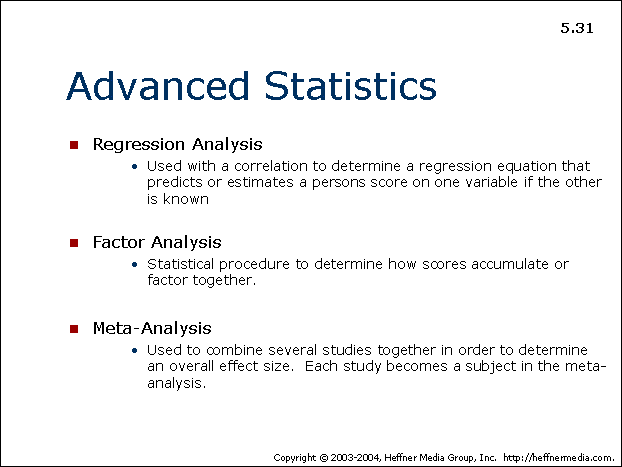advertisement

# 31## Advanced Statistics

• Regression Analysis
• Used with a correlation to determine a regression equation that predicts or estimates a persons score on one variable if the other is known
• Factor Analysis
• Statistical procedure to determine how scores accumulate or factor together.
• Meta-Analysis
• Used to combine several studies together in order to determine an overall effect size. Each study becomes a subject in the meta-analysis.